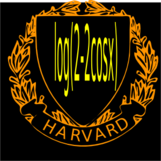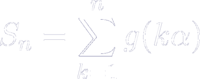Birkhoff sum project 2010
Research project
Birkhoff Sums Over the Golden Rotation
Office: SciCtr 434
 Update posted November 2016: Some notes [PDF] and Slides [PDF] to a talk given at BU in February 2015. In this project with Folkert Tangerman from Stony Brook we look at the Birkhoff sumwith g(x) = 2 log(2 sin(pi x)), where alpha = (sqrt(5)-1)/2 is the golden mean. In a project of 2008 we showed that Sn/log(n)2 is bounded. In the current work, we relate the boundedness of Sn/log(n) to a limiting function f(x) obtained as a limiting difference between consecutive continued fraction expansions. While the function sn(x) = S[x qn](alpha)/log(qn) only converges weakly, the sequence of functions ```fn(x) = S[x qn](pn+1/qn+1) - S[x qn](pn/qn) ``` converges pointwise to a function f(x) on [0,1]. This project started during spring break 2010. Folkert visited Cambridge once in April. Otherwise we worked electronically or by phone. The paper got finalized during a meeting in New London (MA) on May 24, 2010.